# Circumference and Area of a Circle Online Quiz

Following quiz provides Multiple Choice Questions (MCQs) related to Circumference and Area of a Circle. You will have to read all the given answers and click over the correct answer. If you are not sure about the answer then you can check the answer using Show Answer button. You can use Next Quiz button to check new set of questions in the quiz.Q 1 - Find the area and circumference of following circle. Use π = 3.14### Explanation

Step 1:

Given diameter = 20 ; radius r = 20/2 = 10 units

Step 2:

Circumference of Circle, C = 2πr = 2(3.14)10 = 62.8 units

Step 3:

Area of Circle, A = πr2 = (3.14)(10)(10) = 314 square units

Q 2 - Find the area and circumference of following circle. Use π = 3.14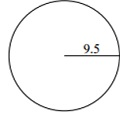### Explanation

Step 1:

Given radius r = 9.5 units

Step 2:

Circumference of Circle, C = 2πr = 2(3.14)9.5 = 59.66 units

Step 3:

Area of Circle, A = πr2 = (3.14)(9.5)(9.5) = 283.39 square units

Q 3 - Find the area and circumference of following circle. Use π = 3.14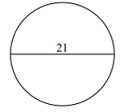### Explanation

Step 1:

Given diameter = 21 ; radius r = 21/2 = 10.5 units

Step 2:

Circumference of Circle, C = 2πr = 2(3.14)10.5 = 65.94 units

Step 3:

Area of Circle, A = πr2 = (3.14)(10.5)(10.5) = 346.19 square units

Q 4 - Find the area and circumference of following circle. Use π = 3.14### Explanation

Step 1:

Given radius r = 5.5 units

Step 2:

Circumference of Circle, C = 2πr = 2(3.14)5.5 = 34.54 units

Step 3:

Area of Circle, A = πr2 = (3.14)(5.5)(5.5) = 94.99 square units

Q 5 - Find the area and circumference of following circle. Use π = 3.14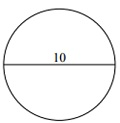### Explanation

Step 1:

Given diameter = 10 ; radius r = 10/2 = 5 units

Step 2:

Circumference of Circle, C = 2πr = 2(3.14)5 = 31.4 units

Step 3:

Area of Circle, A = πr2 = (3.14)(5)(5) = 78.5 square units

Q 6 - Find the area and circumference of following circle. Use π = 3.14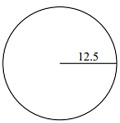### Explanation

Step 1:

Given radius r = 12.5 units

Step 2:

Circumference of Circle, C = 2πr = 2(3.14)12.5 = 78.5 units

Step 3:

Area of Circle, A = πr2 = (3.14)(12.5)(12.5) = 490.63 square units

Q 7 - Find the area and circumference of following circle. Use π = 3.14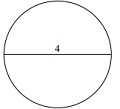### Explanation

Step 1:

Given diameter = 4 ; radius r = 4/2 = 2 units

Step 2:

Circumference of Circle, C = 2πr = 2(3.14)2 = 12.56 units

Step 3:

Area of Circle, A = πr2 = (3.14)(2)(2) = 12.56 square units

Q 8 - Find the area and circumference of following circle. Use π = 3.14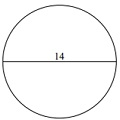### Explanation

Step 1:

Given diameter = 14 ; radius r = 14/2 = 7 units

Step 2:

Circumference of Circle, C = 2πr = 2(3.14)7 = 43.96 units

Step 3:

Area of Circle, A = πr2 = (3.14)(7)(7)= 153.86 square units

Q 9 - Find the area and circumference of following circle. Use π = 3.14### Explanation

Step 1:

Given diameter = 18 ; radius r = 18/2 = 9 units

Step 2:

Circumference of Circle, C = 2πr = 2(3.14)9 = 56.52 units

Step 3:

Area of Circle, A = πr2 = (3.14)(9)(9) = 254.34 square units

Q 10 - Find the area and circumference of following circle. Use π = 3.14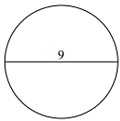### Explanation

Step 1:

Given diameter = 9 ; radius r = 9/2 = 4.5 units

Step 2:

Circumference of Circle, C = 2πr = 2(3.14)4.5 = 28.26 units

Step 3:

Area of Circle, A = πr2 = (3.14)(4.5)(4.5) = 63.59 square units

circumference_and_area_of_a_circle.htm